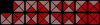# GMLDirectional Grid-Based Collisions

#### ShazzyZang

##### Member
GM Version: 2.3
Target Platform: ALL

Summary:
Using a tileset we will create a grid-based collision detection system.
Each cell will be solid from no directions, all directions, or somewhere in between.
This does require you to move only on full pixels.

Tutorial:

First things first: we need some way to represent what sides of a tile are solid.
The tricky bit here may be storing all that information into a single number (a tile-index).
Binary is a number system that uses only 0 and 1 (instead of decimal, the 0-9 that we use in daily life).

In the decimal system, each digit represents some multiple of 10 (10s, 100s, 1000s, etc).
In the binary system, each digit represents some multiple of 2 (2, 4, 8, 16, etc).

Lets take a look at the number 14. In binary this would be 1110. Starting at the rightmost side:
0 x (2 ^ 0) = 0
1 x (2 ^ 1) = 2
1 x (2 ^ 2) = 4
1 x (2 ^ 3) = 8
If we add these up (0 + 2 + 4 + 8) you'll notice we get 14. This is roughly speaking how binary works.
We're going to treat each direction as a bit in a binary number using the legend below .
BIT 0 : Left
BIT 1 : Down
BIT 2 : Right
BIT 3 : Up

But why would we do this?
Well for every possible combination of solid directions, we will generate a unique number between 0 and 15.
Then we can map each of these combinations to a tile in a tileset:In the above image the dark red is a solid side (and the lighter side is non-solid).
The first image (index 0) has no collisions (as all bits are 0).
The second image (index 1) has 1 collision on the left side. (as the only non-zero bit would be BIT 0).
This continues for all tiles up to and including the last one. This is index 15 (which is all 1's in binary).

Now that we have the collision data represented by the tiles themselves, we need to actually check for collisions.
For this we use the following two functions. While they may be a little large, I've done my best to comment through them.

Code:
``````// Check if any collisions occur at the rectangular position
function collisionCheckRectangle(left, top, right, bottom)
{
// Get information about our collision layer
var layerID = layer_get_id("CollisionLayer");
var tilemapID = layer_tilemap_get_id(layerID);
var tileWidth = tilemap_get_tile_width(tilemapID);
var tileHeight = tilemap_get_tile_height(tilemapID);

// Calculate the corners of the rectangle
// ... c1 = column1, c2 = column2
// ... r1 = row1, r2 = row2
var c1 = (left div tileWidth);
var r1 = (top div tileHeight);
var c2 = (right div tileWidth);
var r2 = (bottom div tileHeight);

// Flags are basically a running total of collisions we've come across
// ... Visit each cell in the rectangle and add there collisions to this 'sum of collisions'
var flags = 0;
for (var c=c1; c<=c2; c++)
{
for (var r=r1; r<=r2; r++)
{
// The |= operation basically compares two binary numbers (our flags and the current tile)
// ... In each spot that -EITHER- of them have a 1, the resulting number has a 1 in that spot too
flags |= tilemap_get(tilemapID, c, r);
}
}
return flags;
}

// Check for collisions relative to your current position
// ... Only consider collisions in the direction you're moving
// ... Ignore pre-existing collisions
// ... dx: the distance to travel along the x-axis
// ... dy: the distance to travel along the y-axis
function collisionCheckRelative(dx, dy)
{
// Here we're going to convert the direction we're moving into a 4-binary-digit flag (like we did with the tiles)
// ... Ex. If we're moving right the resulting binary will be 0001
var dir = point_direction(0, 0, dx, dy);
var flagsDirectional = (1 << (dir div 90));

// Using the function we created before, we get what collisions are occuring at our current position
var flagsCurrent = collisionCheckRectangle(bbox_left, bbox_top, bbox_right, bbox_bottom);

// Using the function we created before, we get what collisions would occur where we want to move
var flagsTarget = collisionCheckRectangle(bbox_left + dx, bbox_top + dy, bbox_right + dx, bbox_bottom + dy);

// This is a little tricky.  First we'll look at the right side of this line.
// ... Flags directional & flagsTarget will return a binary number that has 1's where both numbers had 1s
// ...... That is to say that 0001 & 1111 would produce 0001. This is us ignoring collisions that are not relevant to the direction we're travelling.

// ... The first part then uses ~flagsCurrent. What this means is it flips the bits (anywhere there was a 0, there is now a 1)
// ...... This will produce a binary number that consists only of collisions we don't yet have.
// ...... Since we are &'ing this to the second half of the line, this means that we will be left with collisions at the target location that we did not previously have.

return (~flagsCurrent & (flagsDirectional & flagsTarget));
}``````
And we're done! Now when you want to check for collisions, you simply need to call collisionCheckRelative(dx, dy).